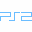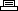Site SearchDef Jam VendettaPrinter Friendly Version

```Cheat mode:
Select battle mode and choose any type of match.
Enter a User ID at the "User Setups" menu. Hold
L1 + L2 + R1 + R2 at the character selection screen
and quickly enter one of the following codes to
unlock the corresponding person.

Arii:
Hold L1 + L2 + R1 + R2 at the character selection
screen and quickly press X, Square, Triangle, Circle,
Square.

Briggs (alternate costume):
Hold L1 + L2 + R1 + R2 at the character selection
screen and quickly press X, Triangle., Circle,
Square, Circle.

Briggs (alternate costume):
Hold L1 + L2 + R1 + R2 at the character selection
screen and quickly press X, Triangle, Square, X,
Circle.

Carla:
Hold L1 + L2 + R1 + R2 at the character selection
screen and quickly press X, Square, X(3).

Chukklez:
Hold L1 + L2 + R1 + R2 at the character selection
screen and quickly press Square(2), Triangle, X,
Circle.

Cruz:
Hold L1 + L2 + R1 + R2 at the character selection
screen and quickly press Circle, Triangle, X(2),
Circle.

D-Mob:
Hold L1 + L2 + R1 + R2 at the character selection
screen and quickly press Square, Triangle, Circle, X,
Circle.

D-Mob (alternate costume):
Hold L1 + L2 + R1 + R2 at the character selection
screen and quickly press Square(2), Triangle,
Square(2).

Dan G:
Hold L1 + L2 + R1 + R2 at the character selection
screen and quickly press X, Circle, X, Circle,
Square.

Deebo:
Hold L1 + L2 + R1 + R2 at the character selection
screen and quickly press Circle(2), X(2), Triangle.

Deja:
Hold L1 + L2 + R1 + R2 at the character selection
screen and quickly press Circle, Square, Circle(2),
X.

DMX:
Hold L1 + L2 + R1 + R2 at the character selection
screen and quickly press Circle, X, Circle, Triangle,
Square.

Drake:
Hold L1 + L2 + R1 + R2 at the character selection
screen and quickly press Triangle, Square, Circle,
X(2).

Funkmaster Flex:
Hold L1 + L2 + R1 + R2 at the character selection
screen and quickly press Circle, Triangle, Circle(2),
Square.

Hold L1 + L2 + R1 + R2 at the character selection
screen and quickly press Triangle(3), Square, Circle.

House:
Hold L1 + L2 + R1 + R2 at the character selection
screen and quickly press Triangle, X, Triangle,
Circle, X.

Iceberg:
Hold L1 + L2 + R1 + R2 at the character selection
screen and quickly press Square, Triangle, Circle,
Square, Circle.

Ludacris:
Hold L1 + L2 + R1 + R2 at the character selection
screen and quickly press Circle(3), Square, Triangle.

Manny (alternate costume):
Hold L1 + L2 + R1 + R2 at the character selection
screen and quickly press Circle, Square, Circle,
Square, Circle.

Masa:
Hold L1 + L2 + R1 + R2 at the character selection
screen and quickly press X, Circle, Triangle, Square(2).

Method Man:
Hold L1 + L2 + R1 + R2 at the character selection
screen and quickly press Square, Circle, X, Triangle,
Circle.

Moses:
Hold L1 + L2 + R1 + R2 at the character selection
screen and quickly press Triangle(2), Square(2), X.

N.O.R.E.:
Hold L1 + L2 + R1 + R2 at the character selection
screen and quickly press Circle, Square, Triangle,
X, Circle.

Nyne:
Hold L1 + L2 + R1 + R2 at the character selection
screen and quickly press Square, Circle, X(2), Triangle.

Omar:
Hold L1 + L2 + R1 + R2 at the character selection
screen and quickly press Circle(2), Square, Triangle(2).

Opal:
Hold L1 + L2 + R1 + R2 at the character selection
screen and quickly press Circle(2), Square(2), Triangle.

Peewee:
Hold L1 + L2 + R1 + R2 at the character selection
screen and quickly press X(2), Square, Triangle, Square.

Peewee (alternate costume):
Hold L1 + L2 + R1 + R2 at the character selection
screen and quickly press X, Triangle(2), Square, Circle.

Penny:
Hold L1 + L2 + R1 + R2 at the character selection
screen and quickly press X(3), Triangle, Circle.

Pockets:
Hold L1 + L2 + R1 + R2 at the character selection
screen and quickly press Triangle, Square, Circle,
Square, X.

Proof (alternate costume):
Hold L1 + L2 + R1 + R2 at the character selection screen
and quickly press X, Square, Triangle, Square, Circle.

Razor:
Hold L1 + L2 + R1 + R2 at the character selection screen
and quickly press Triangle, Square, Triangle, Circle, X.

Razor (alternate costume):
Hold L1 + L2 + R1 + R2 at the character selection screen
and quickly press Square, Circle, X, Triangle(2).

Redman:
Hold L1 + L2 + R1 + R2 at the character selection screen
and quickly press Circle(2), Triangle, Square, X.

Ruffneck:
Hold L1 + L2 + R1 + R2 at the character selection screen
and quickly press X, Square, X, Triangle, Circle.

Ruffneck (alternate costume):
Hold L1 + L2 + R1 + R2 at the character selection screen
and quickly press Square, Circle, Triangle, X, Square.

Scarface:
Hold L1 + L2 + R1 + R2 at the character selection screen
and quickly press Circle, Square, X, Triangle, Square.

Sketch:
Hold L1 + L2 + R1 + R2 at the character selection screen
and quickly press Triangle(2), Circle, Square, X.

Snowman:
Hold L1 + L2 + R1 + R2 at the character selection screen
and quickly press Triangle(2), X(2), Circle.

Spider (alternate costume):
Hold L1 + L2 + R1 + R2 at the character selection screen
and quickly press Square, Triangle, X, Square, Circle.

Spider (alternate costume):
Hold L1 + L2 + R1 + R2 at the character selection screen
and quickly press Circle(2), Square, X, Triangle.

Steel:
Hold L1 + L2 + R1 + R2 at the character selection screen
and quickly press X, Triangle, Circle(2), Triangle.

Tank (alternate costume):
Hold L1 + L2 + R1 + R2 at the character selection screen
and quickly press X, Triangle(2), Circle(2).

T'ai:
Hold L1 + L2 + R1 + R2 at the character selection screen
and quickly press Circle(2), Square, X, Circle.

Zaheer:
Hold L1 + L2 + R1 + R2 at the character selection screen
and quickly press Triangle(2), Square, X(2).

Bounty Club stage:
Hold L1 + L2 + R1 + R2 at the stage selection screen and
quickly press X, Square(2), Circle(2).

Club Luda stage:
Hold L1 + L2 + R1 + R2 at the stage selection screen and
quickly press Circle, Triangle, Square, X, Square.

Def Jam stage:
Hold L1 + L2 + R1 + R2 at the stage selection screen and
quickly press Circle, X, Square, X, Triangle.

Def Jam Vendetta stage:
Hold L1 + L2 + R1 + R2 at the stage selection screen and
quickly press Triangle, Circle, X, Square(2).

Dragon House stage:
Hold L1 + L2 + R1 + R2 at the stage selection screen and
quickly press Triangle, X, Square, X, Circle.

Face Club After Hours stage:
Hold L1 + L2 + R1 + R2 at the stage selection screen and
quickly press Square, X, Square(2), Circle.

Grimeyville stage:
Hold L1 + L2 + R1 + R2 at the stage selection screen and
quickly press Square(3), X, Square.

Warehouse stage:
Hold L1 + L2 + R1 + R2 at the stage selection screen and
quickly press X, X, Square, X, Square.

X's Junkyard stage:
Hold L1 + L2 + R1 + R2 at the stage selection screen and
quickly press X, Square, Square, X, Triangle.
```